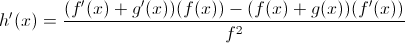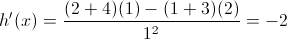# AP Calculus AB : Corresponding characteristics of the graphs of ƒ, ƒ', and ƒ''

## Example Questions

### Example Question #1 : Corresponding Characteristics Of The Graphs Of ƒ, ƒ', And ƒ''

On what intervals is the function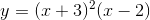both concave up and decreasing?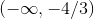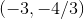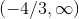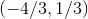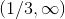Explanation:

The question is asking when the derivative is negative and the second derivative is positive. First, taking the derivative, we get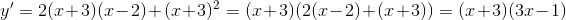Solving for the zero's, we see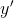hits zero at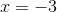and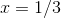. Constructing an interval test,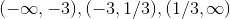, we want to know the sign's in each of these intervals. Thus, we pick a value in each of the intervals and plug it into the derivative to see if it's negative or positive. We've chosen -5, 0, and 1 to be our three values.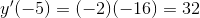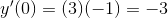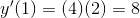Thus, we can see that the derivative is only negative on the interval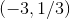.

Repeating the process for the second derivative,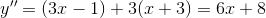The reader can verify that this equation hits 0 at -4/3. Thus, the intervals to test for the second derivative are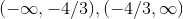.  Plugging in -2 and 0, we can see that the first interval is negative and the second is positive.

Because we want the interval where the second derivative is positive and the first derivative is negative, we need to take the intersection or overlap of the two intervals we got: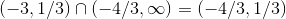If this step is confusing, try drawing it out on a number line -- the first interval is from -3 to 1/3, the second from -4/3 to infinity. They only overlap on the smaller interval of -4/3 to 1/3.

Thus, our final answer is### Example Question #2 : Corresponding Characteristics Of The Graphs Of ƒ, ƒ', And ƒ''

On a closed interval, the function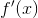is decreasing. What can we say aboutand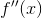on these intervals?is decreasingis decreasing

Two or more of the other answers are correct.is negativeis negativeis negative

Explanation:

Ifis decreasing, then its derivative is negative. The derivative ofis, so this is telling us thatis negative.

Forto be decreasing,would have to be negative, which we don't know.being negative has nothing to do with its slope.

Forto be decreasing, its derivative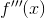would need to be negative, or, alternativelywould have to be concave down, which we don't know.

Thus, the only correct answer is thatis negative.

### Example Question #3 : Corresponding Characteristics Of The Graphs Of ƒ, ƒ', And ƒ''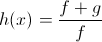If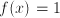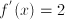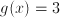and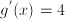,

then find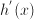.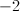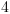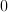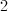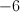Explanation:

We see the answer iswhen we use the product rule.